# 2.E: Exercises

$$\newcommand{\vecs}{\overset { \rightharpoonup} {\mathbf{#1}} }$$ $$\newcommand{\vecd}{\overset{-\!-\!\rightharpoonup}{\vphantom{a}\smash {#1}}}$$$$\newcommand{\id}{\mathrm{id}}$$ $$\newcommand{\Span}{\mathrm{span}}$$ $$\newcommand{\kernel}{\mathrm{null}\,}$$ $$\newcommand{\range}{\mathrm{range}\,}$$ $$\newcommand{\RealPart}{\mathrm{Re}}$$ $$\newcommand{\ImaginaryPart}{\mathrm{Im}}$$ $$\newcommand{\Argument}{\mathrm{Arg}}$$ $$\newcommand{\norm}{\| #1 \|}$$ $$\newcommand{\inner}{\langle #1, #2 \rangle}$$ $$\newcommand{\Span}{\mathrm{span}}$$ $$\newcommand{\id}{\mathrm{id}}$$ $$\newcommand{\Span}{\mathrm{span}}$$ $$\newcommand{\kernel}{\mathrm{null}\,}$$ $$\newcommand{\range}{\mathrm{range}\,}$$ $$\newcommand{\RealPart}{\mathrm{Re}}$$ $$\newcommand{\ImaginaryPart}{\mathrm{Im}}$$ $$\newcommand{\Argument}{\mathrm{Arg}}$$ $$\newcommand{\norm}{\| #1 \|}$$ $$\newcommand{\inner}{\langle #1, #2 \rangle}$$ $$\newcommand{\Span}{\mathrm{span}}$$$$\newcommand{\AA}{\unicode[.8,0]{x212B}}$$

## Exercise $$\PageIndex{1}$$: Equivalence relation

Determine whether or not each of the following binary relations $$R$$ on the given set $$A$$ is reflexive, symmetric,
antisymmetric, or transitive. If a relation has a certain property, prove this is so; otherwise, provide a counterexample
to show that it does not. If $$R$$ is an equivalence relation, describe the equivalence classes of $$A$$.

1. Define a relation $$R$$ on $$A={\mathbb Z}$$ by $$a \,R\, b$$ if and only if $$4 \mid (3a+b).$$
2. Define a relation $$R$$ on $$A={\mathbb Z}$$ by $$a \, R \, b$$ if and only if $$3 \mid (a^2-b^2).$$
3. Let $$A=\mathbb{R}$$, If $$a.b \in \mathbb{R}$$, define $$a \, R \, b$$ if and only if $$a-b \in \mathbb{Z}.$$
4. Define a relation $$R$$ on the set $${\mathbb Z} \times{\mathbb Z}$$ by: $$(a,b)\, R \,(c,d) \mbox{ if and only if } ac=bd.$$
5. Define a relation $$R$$ on $${\mathbb Z}$$ by: $$a R b$$ if and only if $$5 \mid 2a+3b.$$
6. Define a relation $$R$$ on $${\mathbb Z}$$ by: $$a R b$$ if and only if $$2 \mid a^2+b.$$

1.  i.    We shall show that $$R$$  is reflexive on $$A$$.

Proof:

Let $$a \in {\mathbb Z}.$$

Since $$3a+a=4a$$, $$4 \mid (3a+a).$$ Thus $$a R a$$.

Hence  is reflexive on $${\mathbb Z}$$ .

ii.    We shall show that   $$R$$  is symmetric on $$A$$.

Proof:

Let $$a, b \in {\mathbb Z}$$, such that $$a R b$$.

Then $$4 \mid (3a+b)$$. Thus $$3a+b=4m$$, for some  integer $$m.$$

We shall show that  $$b R a$$. That means $$4 \mid (3a+b).$$

Consider  $$3a+b=4m$$

$$(3a+b)+(3b+a)=4m +(3b+a)$$

$$(4b+4a)=4m +(3b+a)$$

$$3b+a=-4m +4b+4a=4(-m+b+a).$$

Since $$m+b+a \in {\mathbb Z}, 4 \mid (3a+b).$$

Hence $$R$$ is symmetric on $${\mathbb Z}.$$

iii.    We shall show that $$R$$ is not antisymmetric on $${\mathbb Z}.$$

Counterexample:

Choose $$a=4$$ and $$b=0$$  .

Then $$3(4)+0=12$$ and  $$4 \mid 12$$, so $$4 \mid 3a+b.$$ Also $$3(0)+4=12$$ and  $$4 \mid 4$$, so $$4 \mid 3b+a.$$

However, $$4 \ne 0$$ , so $$a \ne b$$

Hence $$R$$ is not antisymmetric on $${\mathbb Z}.$$

iv.    We shall show that  $$R$$  is transitive on  $${\mathbb Z}.$$

Proof:

Let  $$a, b, c \in {\mathbb Z}$$, such that $$a R b$$ and $$b R c$$. That means $$4 \mid 3a+b$$ and  $$4 \mid 3b+c$$.

Since $$4 \mid 3a+b$$,  $$3a+b =4 m$$ for some integer $$m$$ , and Since $$4 \mid 3b+c$$,  $$3b+c =4 n$$, for some  integer $$n$$.

We shall show that $$a R c$$. That is $$4 \mid 3a+c.$$

Consider  $$(3a+b)+(3b+c) =4 m+4n \implies 3a+c+4a+4b=4m+4n \implies 3a+c=4(m+n-a-b).$$

Since $$m+n-a-b \in {\mathbb Z}$$, $$4 \mid 3b+c$$. Thus, $$a R c$$.

Hence $$R$$ is transitive on $${\mathbb Z}.$$

v.     Since $$R$$ is  reflexive, symmetric and reflexive, $$a R b$$ is an equivalence relation on $${\mathbb Z}.$$

Equivalence classes:

$$=\{ x \in {\mathbb Z} \mid x \sim 0 \} = \{ x \in {\mathbb Z} \mid 4 \mid (3x+0) \}= \{ x \in {\mathbb Z} \mid 4m=3x, \text{for some integer } m \}=\{ 0, \pm 4, \pm 8, \cdots \}.$$

$$=\{ x \in {\mathbb Z} \mid x \sim 1 \} = \{ x \in {\mathbb Z} \mid 4 \mid (3x+1) \}= \{ x \in {\mathbb Z} \mid 4m=3x+1, \text{for some integer } m\} =\{ \cdots, -3, 1, 5, \cdots \}.$$

$$=\{ x \in {\mathbb Z} \mid x \sim 2 \} = \{ x \in {\mathbb Z} \mid 4 \mid (3x+2) \}= \{ x \in {\mathbb Z} \mid 4m=3x+2, \text{for some integer } m\} =\{ \cdots, -2, 2, 6, \cdots \}.$$

$$=\{ x \in {\mathbb Z} \mid x \sim 3 \} = \{ x \in {\mathbb Z} \mid 4 \mid (3x+3) \}= \{ x \in {\mathbb Z} \mid 4m=3x+3, \text{for some integer } m\} =\{ \cdots, -1, 3, 7, \cdots \}.$$

6.

Proof of Reflexivity:

We can show this directly or using cases:

Directly:

Since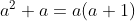, and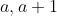are consecutive integers,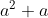is even. Thus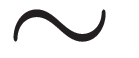is reflexive on.

Proof of Symmetry:

Since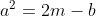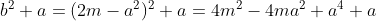.

Thus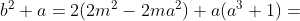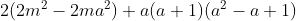.

Since a(a+1) is even,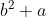is even.

Proof of Transitivity: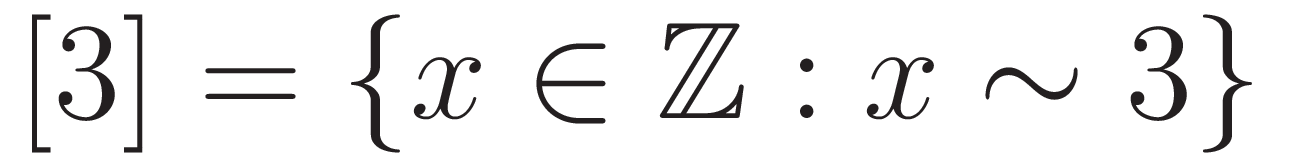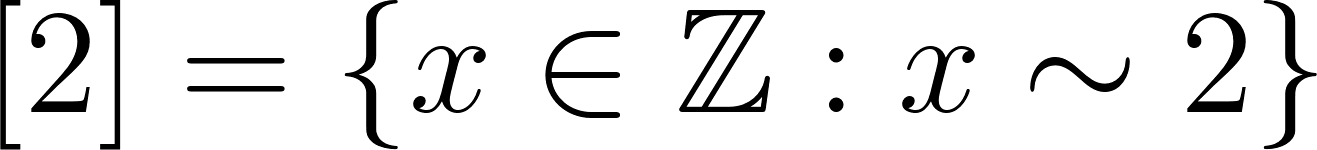## Exercise $$\PageIndex{2}$$: Divides

Let $$a, b,c, d \in \bf Z_+.$$

1. If $$a|b$$ and $$a|c$$, is it necessarily true that $$a|(b + c)?$$
2. If $$a|(b + c)$$, is it necessarily true that $$a|b$$ and $$a|c$$?
3. If $$a|bc$$, is it necessarily true that $$a|b$$ and $$a|c$$?
4. If $$(a+b)|c$$, is it necessarily true that $$a|c$$ and $$b|c$$?
5. If $$a|c$$ and $$b|c$$, is it necessarily true that $$(a+b)|c?$$
6. If $$a^3|b^4$$, is it necessarily true that $$a|b.$$
7. If $$a|b$$, is it necessarily true that $$a^3 \mid b^5?$$
8. If $$c|a$$ and $$d|b$$, is it necessarily true that $$cd|ab$$.

## Exercise $$\PageIndex{3}$$: Divisibility Rules

1. Find all possible values for the missing digit if $$12345X51234$$ is divisible by $$3.$$
2. Using divisibility tests, check if the number $$355581$$ is divisible by $$7$$
3. Using divisibility tests, check if the number $$824112284$$ is divisible by $$5, 4,$$ and $$8.$$

1. An integer (q) is divisible by 3 iff i=0ndiis divisible by 3, where di is the ith digit of q. Thus, the sum of the digits of 12345X51234 must be divisible by 3.

Simplifying, 3 | (30 + X).

Since 3 | 30, 3 must divide X in order to satisfy 3 |12345X51234.

Thus $$X ∈ { 0, 3, 6, or 9}.$$

2.

An integer (q) is divisible by 7 iff 7 | (a - 2b), where b is the last digit of q and a is the remaining digits.

Step 1: a = 35558, b = 1.

Thus, a - 2b = 35556.

Step 2: a = 3555, b = 6.

Thus, a - 2b = 3543.

Note at this point it is clear that 7 ∤ 355581, since 7 ∤ 3543. However, for practice, we will continue on.

Step 3: a = 354, b = 3.

Thus, a - 2b = 348.

Step 4: a = 34, b = 8.

Thus a - 2b = 18.

Since 7 ∤ 18, 7 ∤ 355581.

3.

An integer (q), is divisible by 5 iff its last digits ∈ {0, 5}.

Since 4 ∉ {0, 5}, 5 ∤ 824112284.

An integer (q) is divisible by 8 iff q’s last three digits are divisible by 8.

Since 8 ∤ 284, 8 ∤ 824112284.

An integer (q) is divisible by 4 iff q’s last two digits are divisible by 4.

Since 4 ∣ 84, 4 ∣ 824112284.

## Exercise $$\PageIndex{4}$$: Divisiblity

1. Let $$a$$ be a positive integer such that $$3|(a+1)$$. Show that $$3|(7a+4)$$.

2. Let $$a$$ and $$b$$ be positive integers such that $$7|(a+2b-2)$$ and $$7|(b-9).$$ Prove that $$7|(a+b).$$

3. Let $$a$$, $$b$$, $$c$$ and $$d$$ be positive integers such that $$4|(abc+d)$$, $$4|(adc+b)$$, $$4|(abd+c)$$ and $$4|(bcd+a)$$. Prove that $$4|(a^2+b^2+c^2+d^2).$$

4. Let $$a$$, $$b$$, $$c$$ and $$d$$ be positive integers such that $$ad-bc >1$$. Show that at least of these four integers is not divisible by $$ad-bc$$.

## Exercise $$\PageIndex{5}$$: Middle digit

1. In a $$113$$-digit multiple of $$13$$, the first $$56$$ digits are all $$5$$s and the last $$56$$ digits are all $$8$$s. What is the middle digit?
2. In a $$113$$-digit multiple of $$7$$, the first $$56$$ digits are all $$8$$s and the last $$56$$ digits are all $$1$$s. What is the middle digit?

## Exercise $$\PageIndex{6}$$: True or False

Prove the statements that are true and give counterexamples to disprove those that are false.
Let $$a,b$$ and $$c$$ be integers.

1. If $$a|b$$ then $$b|a$$.
2. If $$a|bc$$ then $$a|b$$ and $$a|c.$$
3. If $$a|b$$ and $$a|c$$ then $$a|bc$$.
4. If $$a|b$$ and $$a|c$$ then $$a|(b+c)$$ and $$a|(b-c)$$.
5. If $$a|(b+c)$$ and $$a|(b-c)$$ then $$a|b$$ and $$a|c$$.
6. If $$a|b$$ and $$a|c$$ then $$a|(b+c)$$ and $$a|(2b+c)$$.
7. If $$a|(b+c)$$ and $$a|(2b+c)$$ then $$a|b$$ and $$a|c$$

## Exercise $$\PageIndex{7}$$: Induction

Prove that for all integers $$n\geq 1, \,5^{2n}-2^{5n}$$ is divisible by $$7.$$

use induction to prove the statement.

## Exercise $$\PageIndex{8}$$: Induction or by cases

Prove that for all integer $$n\geq 1, \,6$$ divides $$n^3-n.$$

use induction to prove the statement.

## Exercise $$\PageIndex{9}$$: True or false

Which of the following statements are true. Prove the statement(s) that are true and give a counterexample for the statement(s) that are false.

If $$a,b,c$$ and $$d$$ are integers such that $$a < b$$ and $$c < d,$$ then $$ac < bd$$.

If $$a,b,c$$ and $$d$$ are integers such that $$a < 0 < b$$ and $$c < 0 < d,$$ then $$ac < bd$$.

If $$a,b,c$$ and $$d$$ are integers such that $$0 < a < b$$ and $$c < 0 < d,$$ then $$ac < bd$$.

If $$a,b,c$$ and $$d$$ are integers such that $$a < b$$ and $$c < d,$$ then $$a-d < b-c.$$

TBD

## Exercise $$\PageIndex{10}$$ Tip?

There are 9 waiters and 1 busboy working at a restaurant. The tips are always in whole number of dollars. At the end of the day, the waiters share the tips equally among themselves as far as possible, each getting a whole number of dollars. The busboy gets what is left. How much does he get on a day when the total amount of the tips is $980. Answer 980 (mod 9) ≡ 8 (mod 9), thus, the busboy would receive$ 8 when the total tip for the day was \$ 980.

## Exercise $$\PageIndex{11}$$ Day of the week

March 1 on some year is a Friday. On what day of the week will Christmas be that year?

Christmas falls on December 25th in any given year. The number of days from March 1 till Christmas (430)+(631) -7=299. Note: We consider March 1 as day zero and there are 6 days from December 25 to December 31, thus seven days must be subtracted from the total number of days. Since 299 = 7(42) + 5 and March 1 is a Friday, Christmas will fall five days after Friday, which is a Wednesday. Thus, if in a given year, March 1 is a Friday, Christmas will fall on a Wednesday that year.

## Exercise $$\PageIndex{12}$$: Inequality

Solve $$0<199m-335<100$$ for all integers $$m$$.

$$m=2$$.